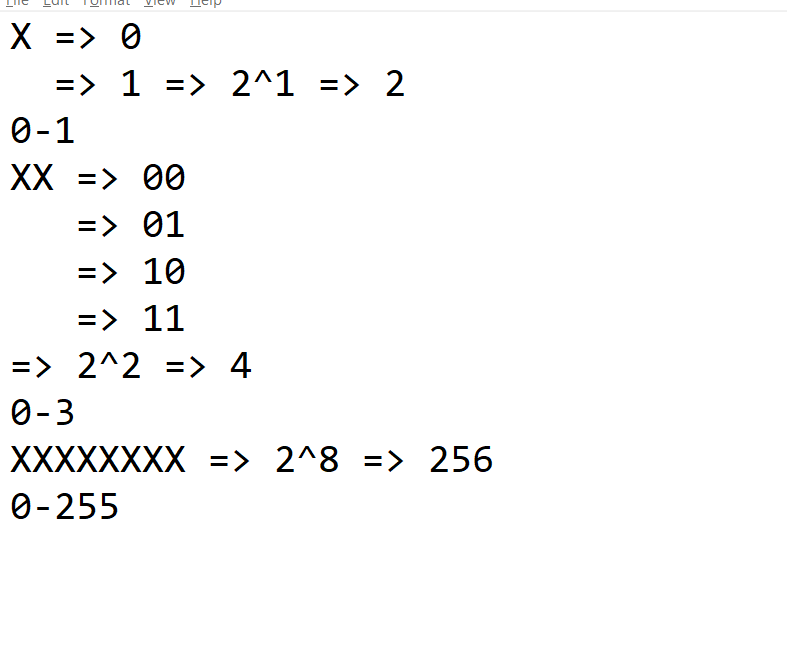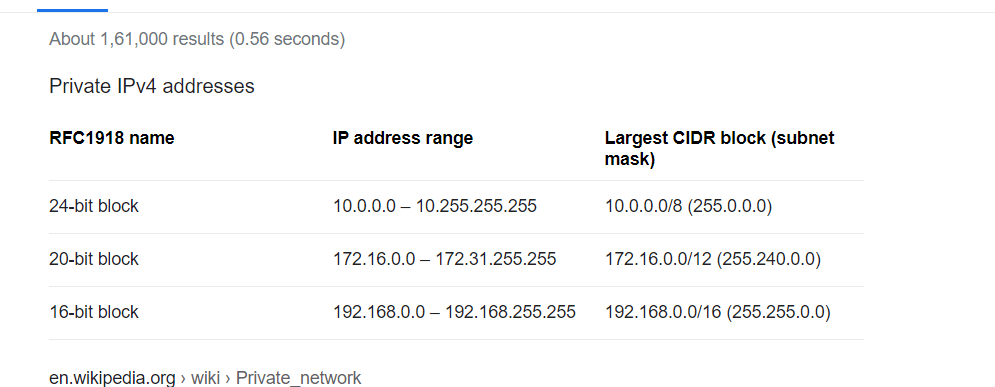### Networking Basics

• We need to create networks to host devices on the network
• Each device on the network recieves an ip address which is unique identifier
• IP address is used for data communication across devices
• Lets fetch ip of the device which we are working on• Network is used to connect multiple devices, so every network will have a limit on number of devices it can connect
• Every network will have a unique network id and every device will have unique host id and this combination of network id and host id is called as IP Address
• Just with ip address we will not be able to determine the network id and host id. To do this wee need subnet mask
``````192.168.0.195
``````
• IpV4 address is 32 bit address divided into 4 octets. Each octet is 8 bits (byte)
``````10.100.10.24
35.12.19.26
``````
• We have 8 positions and in each position we can have 0 or 1
``````XXXXXXXX
each octect will have values from 0 to 255
``````• so the complete ip range is from 0.0.0.0 to 255.255.255.255
• Importance of subnet mask. Subnet mask helps in idenifying network id and host id from ip address
``````ip: 192.168.0.195
sm: 255.255.255.0
nid: 192.168.0
hid: 195

ip: 10.11.0.20
sm: 255.255.0.0
nid: 10.11
hid: 0.20
``````
• In every network we cannot use 2 ip address
• one is all 0 => 192.168.0.0 => network id
• one is all 1 => 192.168.0.255 => broadcast ipaddress
• This approach os subnet masks having 255 or 0 will have 3 possible subnet masks
• 255.255.255.0 => 8 bits for hosts => Size of network => 2^8-1-1 (2^n-2) => 254
• 255.255.0.0 => 16 bits for hosts => 2^16-2 => 65536-2 => 65534
• 255.0.0.0 => 24 bits for hosts => 2^24-2 => 16777216-2 => 16777214
• This approach of creating networks is problematic consider you need to create a network for 2000 devices we need to choose sm 255.255.0.0 => 65534
• This approach is referred as classful ip addressing• So Classless Interdomain routing (CIDR) was introduced and in AWS we use CIDR to define network
• In Classfull we have two possible values for sm octect 0 or 255
• In CIDR we look at subnet mask as binary number
``````ip: 192.168.0.0
sm: 11111111.11111111.11111111.00000000
sm: 255.255.255.0
number of 1's => network id
number of 0's => host
cidr => 192.168.0.0/24
``````
• Lets try out some more cidrs
``````10.10.0.0/23
number of 1's => 23
number of 0's => 32-23 => 9
sm: 11111111.11111111.11111110.00000000
sm: 255.255.254.0
size of network => 2^9-2 => 512-2 => 510
``````
• Lets try to create a network with capacity of 10000 devices
``````2^n-2 ~= 10000
2^n ~= 10000
n = 14 => number of zeros is 14
number of 1's => 32-14 => 18
sm: 11111111.11111111.11000000.00000000
sm: 255.255.192.0
``````
• Lets try to create a network with a capacity of 50000 devices
``````2^n ~= 50000
n = 16 number of zeros =16
number of 1's => 32-16 => 16
sm: 11111111.11111111.00000000.00000000
sm : 255.255.0.0
``````
• Lets try to create a network with capacity of 50 devices
``````2^n ~=50
n = 6
number of 1's => 32-6 => 26
sm: 11111111.11111111.11111111.11000000
sm: 255.255.255.192
``````
• Now while we are creating networks in AWS we create private networks and there are some ip address ranges (cidr ranges) reserved for private networking
• 192.168.0.0/16 => 192.168.0.0 – 192.168.255.255
• 10.0.0.0/8 => 10.0.0.0 – 10.255.255.255
• 172.16.0.0/12 => 172.16.0.0 – 172.31.255.255This site uses Akismet to reduce spam. Learn how your comment data is processed.